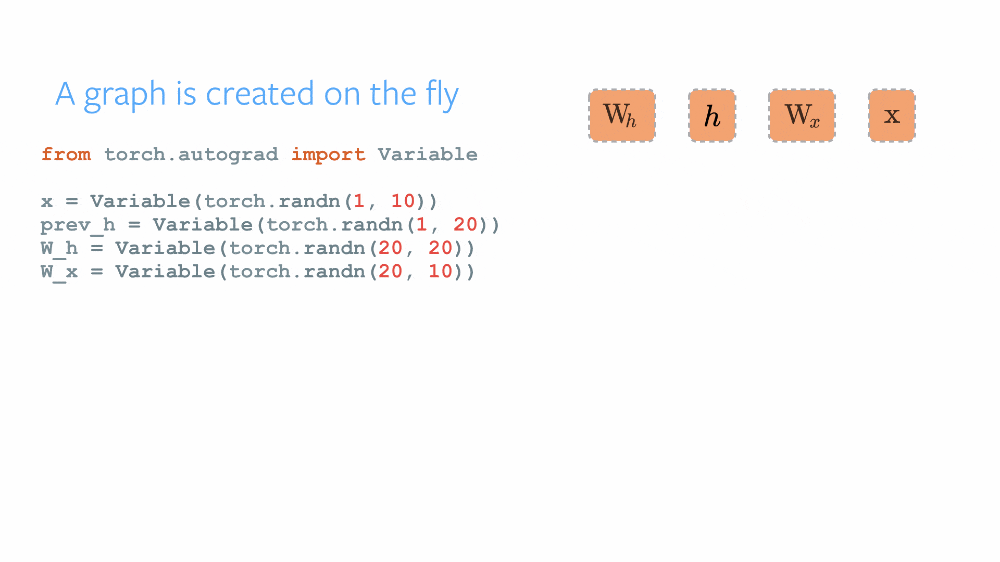# 动态图和静态图## TensorFlow

``````# tensorflow
import tensorflow as tf

first_counter = tf.constant(0)
second_counter = tf.constant(10)
``````
``````def cond(first_counter, second_counter, *args):
return first_counter < second_counter

def body(first_counter, second_counter):
return first_counter, second_counter
``````
``````c1, c2 = tf.while_loop(cond, body, [first_counter, second_counter])
``````
``````with tf.Session() as sess:
counter_1_res, counter_2_res = sess.run([c1, c2])
``````
``````print(counter_1_res)
print(counter_2_res)
``````
``````20
20
``````

## PyTorch

``````# pytorch
import torch
first_counter = torch.Tensor()
second_counter = torch.Tensor()
``````
``````while (first_counter < second_counter):
first_counter += 2
second_counter += 1
``````
``````print(first_counter)
print(second_counter)
``````
`````` 20
[torch.FloatTensor of size 1]

20
[torch.FloatTensor of size 1]
``````